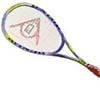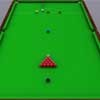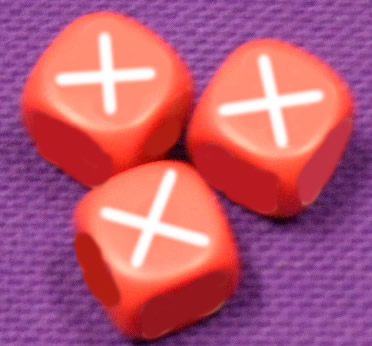#### You may also like### Rain or Shine

Predict future weather using the probability that tomorrow is wet given today is wet and the probability that tomorrow is wet given that today is dry.### Squash

If the score is 8-8 do I have more chance of winning if the winner is the first to reach 9 points or the first to reach 10 points?### Snooker

A player has probability 0.4 of winning a single game. What is his probability of winning a 'best of 15 games' tournament?

# X-dice

##### Age 16 to 18Challenge LevelA new type of 6-sided die, called an X-die, is proposed where instead of the faces being numbered 1 to 6 as usual, the faces are numbered with positive whole numbers such that their sum is 21. In this problem we will say that a die $A$ is worse than a die $B$ if and only if $P(A< B) > P(B< A)$ for a single throw. Conversely, a die $A$ is better than a die $B$ if and only if $P(A< B) < P(B< A)$ for a single throw.

Can you create an X-die which is worse than an ordinary die?

Can you create an X-die which is better than an ordinary die using only the numbers $1$ to $6$ (you don't have to use all of the numbers!)?

Be clear in your explanations or reasoning.

You can prove your results using algebra or explore the problem experimentally using a spreadsheet.

Extension: Explore the notion of a 'worst' or a 'best' X-die